#Function Repository Resource:

# FormatFactorization

Express an integer factorization as it would be seen in a factorization table

Contributed by: Ed Pegg Jr
 ResourceFunction["FormatFactorization"][integer] expresses integer in a factored form as it would be seen in a factorization table.

## Examples

### Basic Examples

Factor various numbers:

 In:=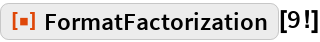Out=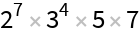In:=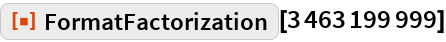Out=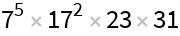In:=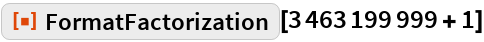Out=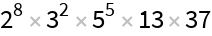In:=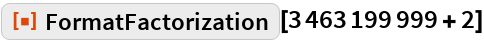Out=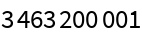### Scope

Make a factorization table:

 In:=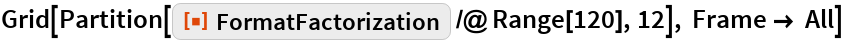Out=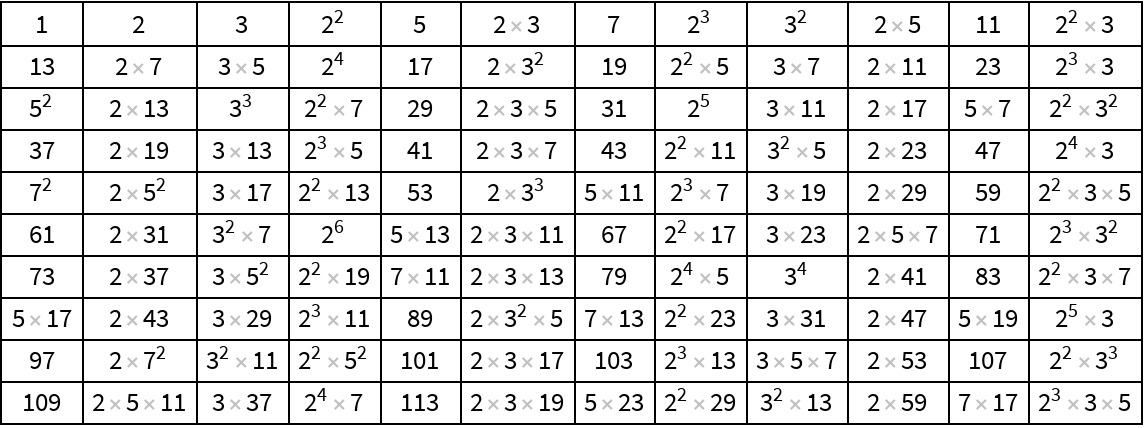## Requirements

Wolfram Language 11.3 (March 2018) or above